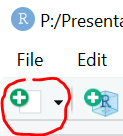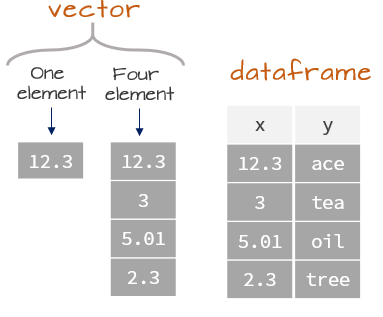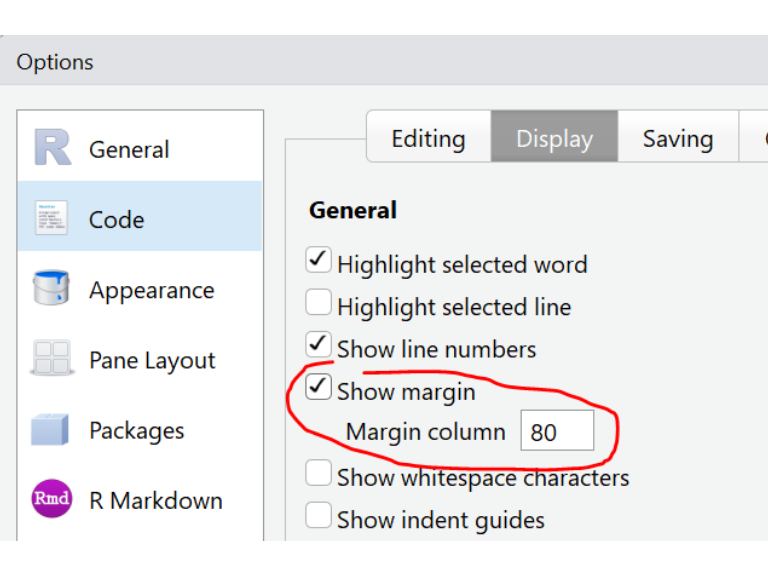## Objects and variables

Data are either explicitly typed in an R command, or they are referenced using variable names (aka objects). For example, the following command performs a simple addition.

2 + 3
 5

The output of 5 is preceded with a bracketed number ( in this example). This is not part of the output value, it’s simply an index used to enumerate individual values if more than one value is generated in the output. This can usually be ignored. We’ll see examples of multi-value outputs later in this exercise.

The above command can be modified by assigning each value to a variable (also referred to as an object in R lingo).

a <- 2
b <- 3
a + b
 5

The objects a and b are each assigned the values 2 and 3 respectively. The combined characters < and - mimic a left arrow indicating that the value to the right is being assigned to the variable to the left. The combined characters ,<-, is referred to as the assignment operator.

Alternatively, you can use the = in lieu of <- however, this is not common practice in the R user community. Furthermore, the left arrow reinforces the idea that the value to the right is being assigned to the variable to the left.

This last example is not the most efficient use of a variable (it’s much easier to type 2 + 3), but most data consist of more than a single value. In this next example, we will sum the values between two sets of numbers (each set consisting of four values).

a <- c(10, 20, 30, 40)
b <- c(1, 2, 3, 4)
a + b
 11 22 33 44

Here, we are assigning more than a single value to variables a and b using the concatenate function c(). Most functions start with the function name followed by parentheses that encompass parameters that are passed to the function. We’ll be making use of many more functions later in this course.

The variables a and b are called vectors in R lingo. Both a and b are said to be four element vectors since they each store 4 values. In the earlier example, a and b were assigned a single value making them one element vectors.

### What name can I assign to a variable?

You are free to assign most any letter or name to a variable as long as it follows these rules:

• does not contain spaces,
• starts with a letter (and not a number),
• contains only alphanumeric characters, underscores _, and dots .,
• is not a reserved word.

You can see a list of reserved words by typing the following at the command line:

?reserved
if, else, repeat, while, function, for, in, next, break,
TRUE, FALSE, NULL, Inf, NaN, NA, NA_integer_, NA_real_, NA_complex, NA_character_

The following are examples of valid variable names:

x
dat1
reg_class_1
dat.l

The following are examples of invalid variable names:

2x
dat ver2
reg%class
abc-d 

Another rule of thumb is to try and avoid creating variable names that match the built-in R function names. For example, we encountered the function c() in the last code chunk. We could create a variable named c if we really wanted to (R will differentiate a variable from a function) as highlighted in the following example:

c <- c(100,200,300,400)
a + b + c
 111 222 333 444

However, such practice is discouraged since it can make code a bit more difficult to read when functions and variable names are intertwined.

## Core data types

There are three core data types in R: numeric (both integer and double), character and logical. You can get an object’s type (also referred to as mode in R) using the typeof() function. Note that R also has a built-in mode() function that will serve the same purpose with the one exception in that it will not distinguish integers from doubles.

### Numeric

The numeric data type is probably the simplest. It consists of numbers such as integers (e.g. whole numbers such as 1 ,-3 ,33 ,0) or doubles (e.g. numbers with a decimal point such as 0.3, 12.4, -0.04, 1.0).

x <- c(1.0, -3.4, 2, 140.1)
typeof(x)
 "double"

Note that removing the fractional part of a number when creating a numeric object does not necessarily create an integer. For example, creating what seems to be an integer object returns double when queried by typeof():

x <- 4
typeof(x)
 "double"

To force R to recognize a value as an integer, add an upper case L to the number.

x <- 4L
typeof(x)
 "integer"

You can also force a double to an integer using the as.integer() function.

x <- 4
x <- as.integer(x)
typeof(x)
 "integer"

### Characters

The character data type consists of letters or words such as "a", "f", "project" and "house value".

x <- "a"
y <- c("a", "ab", "abc")

Characters are always referenced as such using double quotes. Not wrapping character data in double quotes can have unintended consequences:

w <- c(a, b)
w
 10 20 30 40  1  2  3  4

Here, a and b are treated as variable names (they were created earlier in this exercise), so the concatenate function is simply combining the elements in a with those in b.

Numbers can be treated as characters in R.

z <- c("10", "20", "30", "40")

But note that once in character form, numbers cannot be operated on mathematically. For example, the following chunk of code will return an error.

z + z
Error in z + z: non-numeric argument to binary operator

### Logical

Logical values can take on one of two values: TRUE or FALSE. These can also be represented as 1 or 0. For example, to create a logical vector of 4 elements, you can type:

x <- c(TRUE, FALSE, FALSE, TRUE)

or

x <- as.logical(c(1,0,0,1))

Note that in both cases, typeof(x) returns logical. Also note that the 1’s and 0’s in the last example are converted to TRUE’s and FALSE’s internally.

### Coercing from one data type to another

Data can be coerced from one type to another. For example, to coerce the following vector object from character to numeric, use the as.double() function.

y   <- c("23.8", "6", "100.01", "6")
as.double(y)
  23.80   6.00 100.01   6.00

The as.double function forces the vector y to a double (numeric). If you convert y to an integer, R will remove all fractional parts of the number.

as.integer(y)
  23   6 100   6

If the vector contains a non-numeric element, that element is converted to NA. NA is a placeholder for missing values.

y   <- c("23.8", "6", "abc","6")
as.double(y)
 23.8  6.0   NA  6.0

There are many other coercion functions in R, a summary of some the most common ones follows:

Function Purpose
as.character Convert to character
as.numeric() or as.double() Convert to double
as.integer() Convert to integer
as.logical() Convert to a logical

## Command line vs. script file

So far, we’ve run R commands from within an R console or an RStudio command line environment. If you intend on typing more than a few lines of code in a command prompt environment, or if you wish to save a series of commands as part of a project’s analysis, it is probably best that you write and store the commands in an R script file. Such a file is usually saved with a .R extension.

In RStudio, you can create a new script by clicking on the upper left icon, then selecting R script.Create a new script and save it as day01.R in your working folder.

When you type a line of code in your script, the Enter key will not execute the line of code. To run a line of code in an R script, place a cursor anywhere on that line (while being careful not to highlight any subset of that line) and press the shortcut keys Ctrl+Enter on a Windows keyboard or Command+Enter on a Mac.

You can also run an entire block of code by selecting all lines to be run, then pressing the shortcut keys Ctrl+Enter/Command+Enter. Or, you can run the entire R script by pressing Ctrl+Alt+R in Windows or Command+Option+R on a Mac.

## Data structures

Most datasets we work with consist of batches of values such as a table of temperature values or a list of survey results. These batches are stored in R in one of several data structures. These include (atomic) vectors and data frames. Other data structures not explicitly covered in this workshop include matrices and lists.### (Atomic) vector

The atomic vector (or vector for short) is the simplest data structure in R which consists of an ordered set of values of the same type and or class (e.g. numeric, character, etc…). This is the data structure we have worked with thus far. You can think of a vector as a single column of values in a spreadsheet. As such, one important property of a vector is that it cannot mix data types. For example, let’s mix double, integer and character in the vector variable x.

x <- c( 1.2, 5L, "Rt", "2000")

R does not stop us from doing this (if it did, it would have returned an error message). However, if we pass x to the typeof function, we get:

typeof(x)
 "character"

When data types are mixed in a vector, R will convert the element types to the highest common type following the order logical < integer < double < character. In our last example, character is the highest data type in this hierarchy thus forcing all elements in that vector to character.

x
 "1.2"  "5"    "Rt"   "2000"

You can tell that all data elements have been converted to character by the double quotes.

A vector object is an indexable collection of values which allows one to access a specific index number. For example, to access the third element of x, type:

x
 "Rt"

You can also select a subset of elements by index values using the combine function c().

x[c(1,2,4)]
 "1.2"  "5"    "2000"

Or, if you are interested in a range of indexed values such as index 2 through 4, use the sequence, :, operator.

x[2:4]
 "5"    "Rt"   "2000"

### Dataframe

A dataframe is what comes closest to our perception of a data table. You can think of a dataframe as a collection of vector elements where each vector represents a column. As such, it’s important that the vectors have the same number of elements.

name <- c("a1", "a2", "b3")
col1 <- c(23, 4, 12)
col2 <- c(1, 45, 5)
dat  <- data.frame(name, col1, col2)
dat
  name col1 col2
1   a1   23    1
2   a2    4   45
3   b3   12    5

To view each column’s data type we’ll make use of a new function: the structure, str, function.

str(dat)
'data.frame':   3 obs. of  3 variables:
$name: Factor w/ 3 levels "a1","a2","b3": 1 2 3$ col1: num  23 4 12
$col2: num 1 45 5 You’ll notice that the col1 and col2 columns are stored as numeric (i.e. as doubles) and not as integer. There is some inconsistency in R’s characterization of data type. Here, numeric represents double whereas an integer datatype would display integer. For example: col2 <- c(1L, 45L, 5L) dat <- data.frame(name, col1, col2) str(dat) 'data.frame': 3 obs. of 3 variables:$ name: Factor w/ 3 levels "a1","a2","b3": 1 2 3
$col1: num 23 4 12$ col2: int  1 45 5

Data frames can also be constructed without needing to create separate vector objects.

dat  <- data.frame(name = c("a1", "a2", "b3"),
col1 = c(23, 4, 12),
col2 = c(1, 45, 5))
dat
  name col1 col2
1   a1   23    1
2   a2    4   45
3   b3   12    5

Like a vector, elements of a data frame can be accessed by their index (aka subscripts). The first index represents the row number and the second index represents the column number. For example, to list the second row of the third column, type:

dat[2, 3]
 45

If you wish to list all rows for columns one through two, leave the first index blank:

dat[ , 1:2]
  name col1
1   a1   23
2   a2    4
3   b3   12

Or, if you wish to list the third row for all columns, leave the second index blank:

dat[ 3 , ]
  name col1 col2
3   b3   12    5

You can also reference columns by their names if you append the $ character to the dataframe object name. For example, to list the values in the column named col2, type: dat$col2
  1 45  5

To get the column names of a table, use the names() function.

names(dat)
 "name" "col1" "col2"

## R coding style guide

### Spacing

Spaces help improve readability. Add spaces around operators (this includes the assignment operator) and after commas.

Place a space before an open parenthesis/curly brace except when an open parenthesis is preceded with a function name. Place a space after a closed parenthesis/curly brace.

a <- b * 3 a<-b*3
a <- c(2, 4, NA) a<-c(2,4,NA)
(a > 4) | (b < 5) (a>4)|(b<5)
summary(dat1) summary (dat1)
(a == b) ( a == b )
dat[ , 3] dat[,3]

### Parentheses

Use parentheses to isolate conditional statements. Do not wrap overall statements with parentheses.

(a >= b) & (b < c) a >= b & b < c
(a >= b) & (b < c) ((a >= b) & (b < c))

Comments allow the user to document parts of the code without the comments being interpreted by R as code. All comments are preceded by the # character.

# Assign three values to p
p <- c(23, 1.2, 5)

Comments should be used to isolate key steps in a workflow. But they should not be used to document each and every line of code (except when used in an instructional setting).

An empty line should be placed before the comment but not after. A space should separate the first letter of a comment and the # symbol.

# Load file

# Process file
dat$date <- ymd(YY,MM,DD) dat1 <- dat[ , -c(2,3)] #Load file dat <- read.csv("file.csv") #Process file #Create date column dat$date <- ymd(YY,MM,DD)
#Subset columns
dat1 <- dat[ , -c(2,3)]

### Line length

Try to limit the line length to 80 characters. You can add an 80 character vertical line to your code editor via Tools >> Global Options >> Code and the Display tab.A popular (and universal) data file format is the comma separated file format known as a CSV file. To open a csv data table in R, use the read.csv() function. In the next example, we will load registrar’s course schedule for the Spring of 2019. But first, we will need to let our R session know where to find the data file. We’ll make use of RStudio’s interface to specify our working directory. In the menu bar, navigate to Session >> Set Working Directory >> Choose Directory and select the folder where you have the SP1818.csv file saved. Or, if you are familiar with directory structures, you can type the full path in R using the setwd() function as in:

# On Windows ...
setwd("C:/Users/Jdoe/Workshop/Data")

# On Macs ...
setwd("/Users/Jdoe/Workshop/Data")

Next, we’ll open the data file and store its contents in an object we’ll name dat.

dat <- read.csv("SP1819.csv", stringsAsFactors = FALSE)

Now, identify the data types associated with each variable (aka column).

str(dat)
'data.frame':   717 obs. of  13 variables:
$Course : chr "AA118" "AA223" "AA223" "AA231" ...$ Section  : chr  "A" "A" "A" "A" ...
$Cr : chr "2" "4" "4" "4" ...$ Days     : chr  "TR" "TR" "TR" "MW" ...
$Times : chr " 2:30pm- 4:00pm" " 8:00am- 9:30am" " 9:45am-10:45am" "11:00am-12:15pm" ...$ Title    : chr  "Dance Technique Lab: Dance Forms of the African Diaspora: Hip-hop (See TD118)" "Critical Race Feminisms and Tap Dance (See WG223)" "Critical Race Feminisms and Tap Dance (See WG223)" "Caribbean Cultures (See AY231)" ...
$DistReq : chr "" "A" "A" "" ...$ Diversity: chr  " " "U " "U " "I " ...
$Room : chr " " " " " " " " ...$ Reg      : int  0 0 0 0 0 0 0 0 0 0 ...
$Max : int NA NA NA NA NA NA NA NA NA NA ...$ Exam     : int  NA NA NA NA NA NA NA NA NA NA ...
\$ Faculty  : chr  "Akuchu     " "Thomas, S  " "Thomas, S  " "Bhimull    " ...`